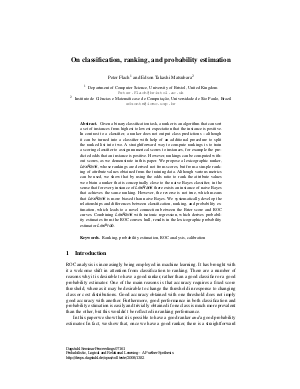Document# On classification, ranking, and probability estimation

### Authors Peter Flach, Edson Matsubara## File

DagSemProc.07161.8.pdf
• Filesize: 285 kB
• 10 pages

## Cite As

Peter Flach and Edson Matsubara. On classification, ranking, and probability estimation. In Probabilistic, Logical and Relational Learning - A Further Synthesis. Dagstuhl Seminar Proceedings, Volume 7161, pp. 1-10, Schloss Dagstuhl - Leibniz-Zentrum für Informatik (2008)
https://doi.org/10.4230/DagSemProc.07161.8

## Abstract

Given a binary classification task, a ranker is an algorithm that can sort a set of instances from highest to lowest expectation that the instance is positive. In contrast to a classifier, a ranker does not output class predictions – although it can be turned into a classifier with help of an additional procedure to split the ranked list into two. A straightforward way to compute rankings is to train a scoring classifier to assign numerical scores to instances, for example the predicted odds that an instance is positive. However, rankings can be computed without scores, as we demonstrate in this paper. We propose a lexicographic ranker, LexRank , whose rankings are derived not from scores, but from a simple ranking of attribute values obtained from the training data. Although various metrics can be used, we show that by using the odds ratio to rank the attribute values we obtain a ranker that is conceptually close to the naive Bayes classifier, in the sense that for every instance of LexRank there exists an instance of naive Bayes that achieves the same ranking. However, the reverse is not true, which means that LexRank is more biased than naive Bayes. We systematically develop the relationships and differences between classification, ranking, and probability estimation, which leads to a novel connection between the Brier score and ROC curves. Combining LexRank with isotonic regression, which derives probability estimates from the ROC convex hull, results in the lexicographic probability estimator LexProb.
##### Keywords
• Ranking
• probability estimation
• ROC analysis
• calibration

## Metrics

• Access Statistics
• Total Accesses (updated on a weekly basis)
0